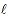##### Estimated Numerical Results and Simulation of the Plant Disease Model Incorporating Wind Strength and Insect Vector at Equilibrium

A. L. M. Murwayi, T. Onyango, B. Owour

Journal of Advances in Mathematics and Computer Science, Page 1-17
DOI: 10.9734/JAMCS/2017/36817

Numerical simulations facilitate in the study of the behaviour of systems whose mathematical models are too complex to obtain analytical solutions. In this paper, we used assumed values of the model parameters and variable to simulate a non-linear deterministic model for plant vector borne diseases developed by Murwayi in . Available models incorperate one climate change parameters at a time but our model includes the three temperature, precipitate and wind at ago. The non linear deterministic model included the climate change parameters with temperature and precipitation that influence the biting rate as (a) while wind as an agent of movement of the insect vector, emmigration and immigration is incorperatedin the model as (±θ). We obtained the basic reproductive number R0 and carried out the normalized sensitivity analysis to obtain the positive and negative numerical sensitivity indices to determine the parameters that have the greatest and lowest impact on the basic reproductive number R0  of the model.  We used Matlab ODE 45 in built solver to simulate the dynamics of the model.

##### The “Vertical” Generalization of the Binary Goldbach’s Conjecture as Applied on “Iterative” Primes with (Recursive) Prime Indexes (i-primeths)

Andrei-Lucian Drăgoi

Journal of Advances in Mathematics and Computer Science, Page 1-32
DOI: 10.9734/JAMCS/2017/36895

This article proposes a synthesized classification of some Goldbach-like conjectures, including those which are “stronger” than the Binary Goldbach’s Conjecture (BGC) and launches a new generalization of BGC briefly called “the Vertical Binary Goldbach’s Conjecture” (VBGC), which is essentially a meta-conjecture, as VBGC states an infinite number of conjectures stronger than BGC, which all apply on “iterative” primes with recursive prime indexes (i-primeths). VBGC was discovered by the author of this paper in 2007 and perfected (by computational verifications) until 2017 by using the arrays of matrices of Goldbach index-partitions, which are a useful tool in studying BGC by focusing on prime indexes. VBGC distinguishes as a very important conjecture of primes, with potential importance in the optimization of the BGC experimental verification (including other possible theoretical and practical applications in mathematics and physics) and a very special self-similar property of the primes set.

##### A Design of a Low-Reynolds Number Airfoil that Leads to the Formation of Separation Bubbles at the Leading Edge

Faith Chelimo Kosgei

Journal of Advances in Mathematics and Computer Science, Page 1-20
DOI: 10.9734/JAMCS/2017/36806

The aerodynamics of airfoils at low Reynolds numbers (Re) has become increasingly important from both fundamental and industrial points of view, due to recent developments in small wind turbines, small-unmanned aerial vehicles (UAVs), micro-air vehicles (MAVs), as well as researches on bird/insect flying aerodynamics. Researchers on airfoil aerodynamics have focused on conventional aircraft design with Re beyond 5×105, where separation bubble forming on the leading edge has positive impact on aerodynamic performance of the airfoil, increasing the lift. Separation bubble not form at low Re (<1.0×104), causing the value of lift to be small. The shape of the airfoil is change to get an airfoil that can cause separation at the leading edge. This is made possible by varying the maximum thickness, maximum camber and position of maximum camber to obtain different airfoils and their pressure difference computed. Reynolds numbers considered here are 1000 to 10, 000.  It is from the study that a desired airfoil is obtained that has high lift and leading edge separation. In this study, it is now possible to design airfoil that can work well at low Reynolds number that is where velocity is low.

##### Stability Analysis of a Motor-Gear-Alternator (MGA) System Using Bode Plot Technique

Wesley Koech

Journal of Advances in Mathematics and Computer Science, Page 1-11
DOI: 10.9734/JAMCS/2017/37241

Koech and the associates designed a Motor-Gear-Alternator (MGA) model and identified the effective parameters of that model. Nonetheless, the stability of the model to determine its applicability is still missing. In this paper, the author attempted to design a stable for Motor-Gear Alternator (MGA) system using Bode plots criterion. The goal was to develop a system that could perform its design and intended functions without any interruptions. Simulation showed that the system is stable for 1 ≤ Gear ratio ≤ 7 and the excess voltage is achieved when the Gear ratio > 3. Moreover, when the Gear ratio is three, the system is stable with good response. This research has contributed to the field of system modeling, system identification and system stability analysis.

##### Properties of T–Anti-Fuzzy Ideals of a 1–Near-Ring

G. Chandrasekaran, B. Chellappa, M. Jeyakumar

Journal of Advances in Mathematics and Computer Science, Page 1-14
DOI: 10.9734/JAMCS/2017/37091

In this paper, we define Anti-fuzzy ideal of a-near-ring in R and T-anti-fuzzy ideal of a-near-ring in R. we made an attempt to study the properties of T-anti-fuzzy ideal of a-near-ring, union of T-anti-fuzzy ideals of-near-ring, join of T-anti-fuzzy ideal of a-near-ring in R, join of a family of T-anti-fuzzy ideal of a-near-ring in R and family of union of T-anti-fuzzy ideal of-near-ring in R.